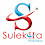# Slow Motion | Bharat | Full Song Piano Notes | Vishal & Shekhar

Song – Slow Motion
Movie – Bharat
Singers – Vishal-Shekhar, Nakash Aziz, Shreya Ghoshal
Musicians – Meghdeep Bose

Song – Slow Motion
Movie – Bharat
Singers – Vishal-Shekhar, Nakash Aziz, Shreya Ghoshal
Label– T-SeriesSlow Motion | Bharat | Full Song Piano Notes | Vishal & Shekhar
Starting Music:-
D+D+F+E+F+  D+E+D+C+D+
[4 TIMES]

Aaj ki shaam lage picture ka scene koyi
D+D+F+E+F+  D+E+D+C+D+
Picture ka scene koyi
A C+D+ D+E+D+C+D+
(Picture ka scene koyi
A C+D+ E+D+C+D+
Picture ka scene koyi

A C+D+ D+E+F+ C+D+)

Main hero jaisa aur tu heroine koyi
D+D+F+E+F+  D+E+D+C+D+
Tu heroine koyi
A C+D+ D+E+D+C+D+
(Tu heroine koyi
A C+D+ E+D+C+D+
Tu heroine koyi

A C+D+ D+E+F+ C+D+)

Story kahan kahan se
D+G+ G+E+D+C+G+
Oh ghoom ghaam ke
E+D+E+ C+C+E+
Aa gayi motion mein
AC+D+F+D+F+E+

Aaja doob jaaun teri aankhon ke ocean mein
D+D+ D+F+E+F+ D+E+D+C+AC+E+E+
Slow motion mein
E+F+E+ D+E+D+ C+E+D+
(Music:- D+D+F+E+F+  D+E+D+C+D+) X2

Aaja doob jaaun teri aankhon ke ocean mein
D+D+ D+F+E+F+ D+E+D+C+AC+E+E+
Slow motion mein
E+F+E+ D+E+D+ C+E+D+
(Music:- D+D+F+E+F+  D+E+D+C+D+) X2

Ring leke bade waali ek din
Tujhe maine kar lena hai win
GG GC+GC+ D+E+ D+ C+

Ring leke bade waali ek din

Tujhe maine kar lena hai win
A GC+ GC+
Babies honge
GC+ GC+
BbBb BbBb AA

Ab ho jaane do ji meeting
Phir hogi humari setting
Phir mummy papa ko bolenge
A GC+ GC+ GC+D+E+
Karo humari wedding

Hoga hill pe honeymoon
F+F+ F+G+F+E+F+E+D+
Delay ismein nahi soon
F+F+ F+G+F+E+F+E+D+
Din thode bache hain
F+F+ F+G+F+E+F+E+D+
Relationship ke promotion mein
E+D+C+ AC+D+F+D+F+E+

Aaja doob jaaun teri aankhon ke ocean mein

D+D+ D+F+E+F+ D+E+D+C+AC+E+E+
Slow motion mein
E+F+E+ D+E+D+ C+E+D+
(Music:- D+D+F+E+F+  D+E+D+C+D+) X2

Aaja doob jaaun teri aankhon ke ocean mein
D+D+ D+F+E+F+ D+E+D+C+AC+E+E+
Slow motion mein
E+F+E+ D+E+D+ C+E+D+
(Music:- D+D+F+E+F+  D+E+D+C+D+) X2

1.2.This comment has been removed by the author.

3.This comment has been removed by the author.

4.thank for this notes.. Punjabi songs lyrics

5.6.Latest New Bhojpuri songs 2020 Play Online new bhojpuri song 2020 mp3 download now and the latest track to join is Bhojpuri superstar Kesari Lal Yadav ,Pawan Singh, Ritesh Pande and More here you can find best and bhojpuri songs 2020

7.